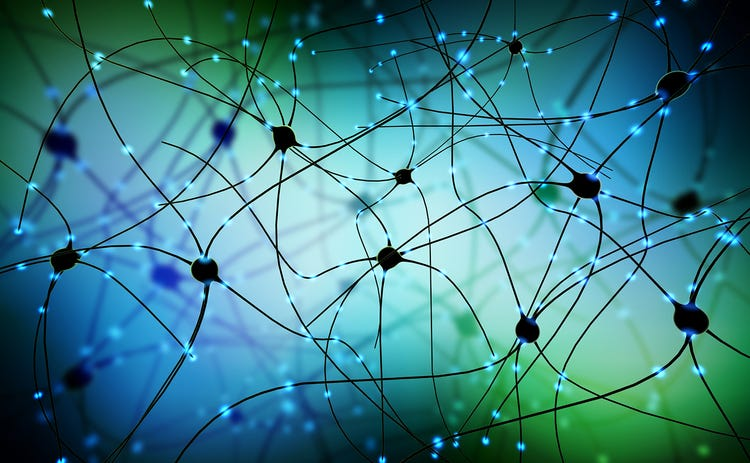# Building a lane detection system

with Python 3 & OpenCV

In this tutorial, we will learn how to build a software pipeline for tracking road lanes using computer vision techniques.

challenges in road lane detection ?

The lines on the road that show us where the lanes are act as our constant reference. We are using canny detector-Hough transform based lane detection.

# SQL Data Base Integration with Python for Data Science

PostgreSQL

In your Data science journey you wont be receiving data in excel sheets. Data will mostly in DB from where we have to extract necessary data. Here we will see SQL data base postgresql and how to access it in your python code for your AI or Data Science activities.

What is a DataBase?

A database is a collection of tables related to each other via columns. For most real-world projects, a database is a must. We can use SQL (Structured Query Language) to create, access, and manipulate data. …

# ResNet

Residual Neural network on CIFAR10

In my previous posts we have gone through

Residual Network (ResNet) is a Convolutional Neural Network (CNN) architecture which can support hundreds or more convolutional layers. ResNet can add many layers with strong performance, while previous architectures had a drop…

# Image Classification With CNN

PyTorch on CIFAR10

In my previous posts we have gone through

Let us try to classify images using Convolution

# Training Deep Neural Networks on a GPU with PyTorch

MNIST using feed forward neural networksIn my previous posts we have gone through

Let us try to by using feed forward neural network on MNIST data set.

Step 1 : Import libraries & Explore the data and data preparation

With necessary libraries imported and data is loaded as pytorch tensor,MNIST data set contains 60000 labelled images. Data is split into training and validation set with 50000 and 10000 respectively with random split.

`val_size = 10000 train_size…`

# Image classification with PyTorch

CIFAR 10 Data set using logistic regression

In my previous posts we have gone through

Let us try to solve image classification of CIFAR-10 data set with Logistic regression.

Step 1 : Import necessary libraries & Explore the data set

We are importing the necessary libraries pandas , numpy , matplotlib ,torch ,torchvision. With basic EDA we could infer that CIFAR-10 data set contains 10 classes of image, with training data set size of 50000 images , test data set size of 10000.Each image…

# Linear Regression with PyTorch

Your first step towards deep learning

In my previous posts we have gone through

Here we will try to solve the classic linear regression problem using pytorch tensors.

1 What is Linear regression ?

y = Ax + B.
A = slope of curve
B = bias (point that intersect y-axis)
y=target variable
x=feature variable

# Tensors — Basics of pytorch programming

We have discussed the concept of deep learning and neural network in our previous post. In this post we will discuss about the basic element of a neural network program which is tensor.

1 What is a Tensor ?

A tensor is the primary data structure of neural network. Mathematical generalization of a tensor can be understood more precisely with the following table.

# Deep Learning — Artificial Neural Network(ANN)

Building your first neural network in less than 30 lines of code.

1.What is Deep Learning ?

Deep learning is that AI function which is able to learn features directly from the data without any human intervention ,where the data can be unstructured and unlabeled.

1.1 Why deep learning?

ML techniques became insufficient as the amount of data is increased. The success of a model heavily relied on feature engineering till last decade where these models fell under the category of Machine learning. Where deep learning models deals with finding these features automatically from the raw data.

1.2 Machine learning…## Arun Purakkatt

MBA,M.Tech-ML Engineer/Data scientist